##### Statistics For Dummies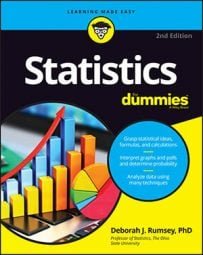By far the most common measure of variation for numerical data in statistics is the standard deviation. The standard deviation measures how concentrated the data are around the mean; the more concentrated, the smaller the standard deviation. It’s not reported nearly as often as it should be, but when it is, you often see it in parentheses, like this: (s = 2.68).

## The formula for the sample standard deviation (s) is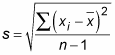where xi is each value in the data set, x-bar is the mean, and n is the number of values in the data set. To calculate s, do the following steps:

1. Calculate the average of the numbers,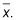2. Subtract the mean from each number (x)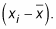3. Square each of the differences,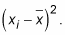4. Add up all of the results from Step 3 to get the sum of squares,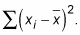5. Divide the sum of squares (found in Step 4) by the number of numbers minus one; that is, (n – 1).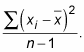6. Take the square root to get the result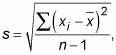7. This is the sample standard deviation, s. Whew!

At the end of Step 5, you have found a statistic called the sample variance, denoted by s2. The variance is another way to measure variation in a data set; its downside is that it’s in square units. If your data are in dollars, for example, the variance would be in square dollars — which makes no sense. That’s why you proceed to Step 6. Standard deviation has the same units as the original data.

## Standard deviation formula example:

Suppose you have four quiz scores: 1, 3, 5, and 7. The mean is 16 ÷ 4 = 4 points. Subtracting the mean from each number, you get (1 – 4) = –3, (3 – 4) = –1, (5 – 4) = +1, and (7 – 4) = +3. Squaring each of these results, you get 9, 1, 1, and 9. Adding these up, the sum is 20. In this example, n = 4, and therefore n – 1 = 3, so you divide 20 by 3 to get 6.67, which is the variance. The units here are “points squared,” which obviously makes no sense. Finally, you take the square root of 6.67, to get 2.58. The standard deviation for these four quiz scores is 2.58 points.

Because calculating the standard deviation involves many steps, in most cases you have a computer calculate it for you. However, knowing how to calculate the standard deviation helps you better interpret this statistic and can help you figure out when the statistic may be wrong.